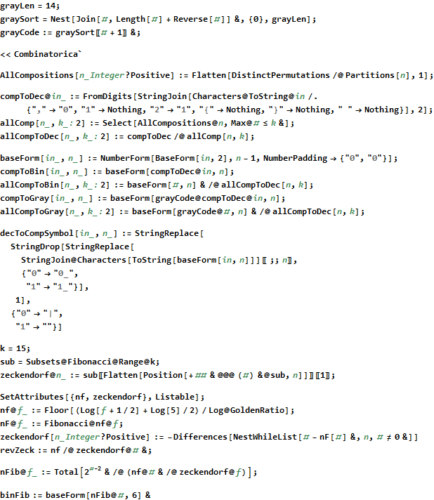# 5-color graph solutions to the Hadwiger–Nelson problem

These are a simple animated visualizations I created while studying the problem. They contain a sequence of 2s frames starting at 130 vertices and incrementing 62. The red numbered text are the first few starting vertex numbers showing the hexagonal structure of the construction. On the left is a list of sorted and color coded vertices by the number of edges on a vertex for that frame’s shown vertices (it also lists in the right column the number of vertices with that number of edges & color). The solution data was obtained from: https://github.com/marijnheule/CNP-SAT

Here is a link to a Mathematica Notebook (50Mb) that has interactive ListAnimated graphs with vertex data (vertex number, symbolic and numeric coordinates, number of edges on the vertex in the frame) in Tooltip on mouseover.

# 3D Visualization of the rays of E6 & E7 in Kochen-specker theory by Ruuge & Waegell/Aravind

In several papers on BKS proofs, Arthur Ruuge’s “Exceptional and Non-Crystallograpic Root Systems and the Kochen-Specker Theorem” https://arxiv.org/abs/0906.2696v1 and Mordecai Waegell & P.K. Aravind’s “Parity proofs of the Kochen-Specker theorem based on the Lie algebra E8” https://arxiv.org/abs/1502.04350v2, in addition to E8, E6 and E7 is studied. Using the visualization developed for my recent paper and prior papers, I present here the related visualizations for E6 and E7 as discussed in those papers.The 72 E6 vertices derived from E8 and projected to 3D using the “E8->H4” basis vectors. The 15*72=1080 edges are shown in the upper left, the 36 anti-podal rays are shown in the upper right along with the hull group vertex counts and norm distance. The bottom image shows the 4 hulls – yellow Icosahedron (12), cyan dodecahedron (20) and the orange/pink pentagonal prisms (40)).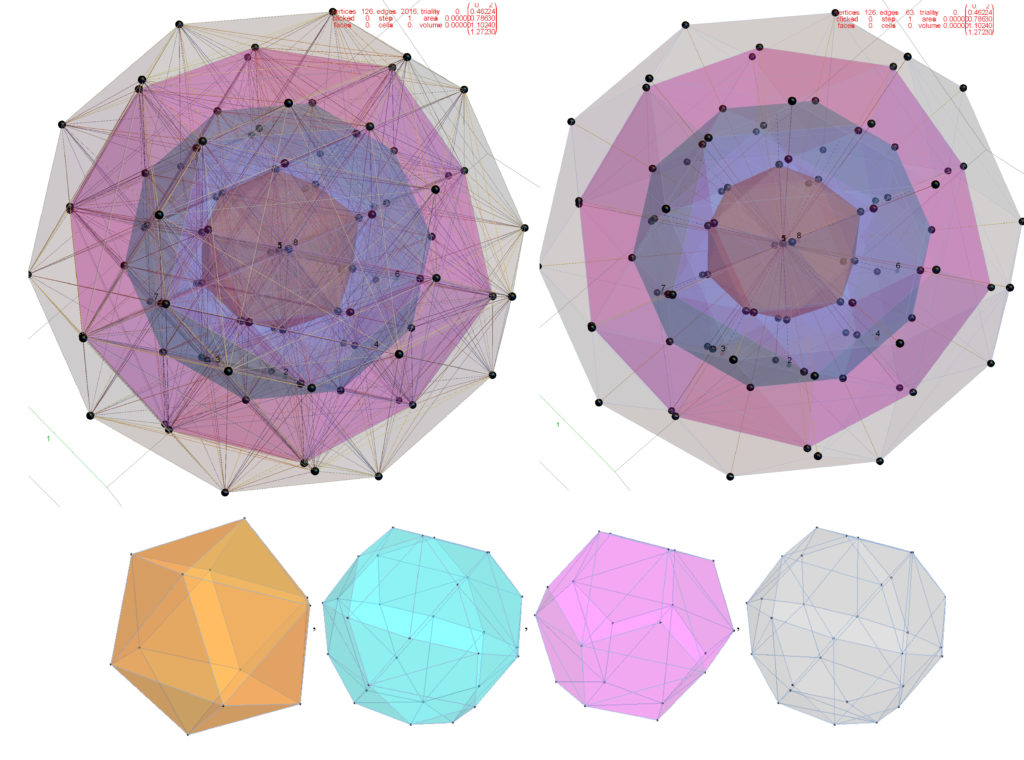The 126 E7 vertices derived from E8 and projected to 3D using the “E8->H4” basis vectors. The 16*126=2016 edges are shown in the upper left, the 63 anti-podal rays are shown in the upper right along with the hull group vertex counts and norm distance. The bottom image shows the 4 hulls – orange 2 overlapping Icosahedrons (24), pink2 overlapping dodecahedron (40), and cyan & gray icosidodecahedrons(60) with 2 vertices at the origin.

# My Latest paper published on Vixra – 3D Polytope Hulls of E8 4_21, 2_41, and 1_42

https://vixra.org/pdf/2005.0200v1.pdf

or also available directly from this website:

https://theoryofeverything.org/TOE/JGM/3D_Polytope_Hulls_of_E8-421-241-142.pdf

Using rows 2 through 4 of a unimodular 8x8 rotation matrix, the vertices of E8 421, 241, and 142 are projected to 3D and then gathered & tallied into groups by the norm of their projected locations. The resulting Platonic and Archimedean solid 3D structures are then used to study E8’s relationship to other research areas, such as sphere packings in Grassmannian spaces, using E8 Eisenstein Theta Series in recent proofs for optimal 8D and 24D sphere packings, nested lattices, and quantum basis critical parity proofs of the Bell-Kochen-Specker (BKS) theorem.

A few new Figures from the paper.

Now for a few new visualizations that are not in the paper…

# 3D visualization of E8 1_42 polytope

This is what I expect to be the first ever 3D visualization of the E8 1_42 polytope with 17280 vertices showing concentric hulls of Platonic solid related structures!E8 1_42 polytope with 17280 vertices showing concentric hulls of Platonic solid related structures! Vertex colors represent the overlap counts.Each of 74 concentric hulls based on 3D Norm’d vertex positions (with varying opacity in sets of 8). Vertex counts in each hull listed above.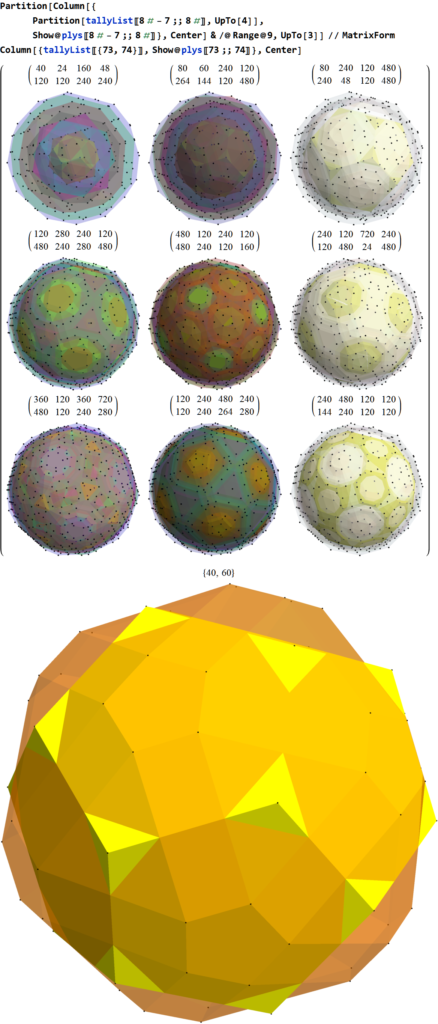9 sets of 8 concentric hulls plus the last 2 outer hulls, Vertex counts in each of 8 hull listed above. Notice this is a combination of two overlapped dodecahedrons (40) and a Nonuniform Rhombicosidodecahedron (60).

For the sake of completeness in visualization, see below for various projections to 2D. Click these links for a higher resolution PNG or the SVG version.

# Nested Lattices of E8 in Complex Projective 4-Space

I read an interesting article about a pattern discovered by Warren D. Smith (discussed at length here):

“The sum of the first three terms in the Eisenstein E_4(q)  Series Integers of the Theta series of the E8 lattice is a perfect fourth power: 1 + 240 + 2160 = 2401 = 7^4”

So I decided to visualize the 2401=1+240+2160 vertex patterns of E8 using my Mathematica codebased toolset based on some previous work I put on my Wikipedia talk page.

The image below represents various projections showing 6720 edges of the 240 E8 vertices, plus a black vertex at the origin, and the 2160 Witting Polytope E8 2 _ 41 vertices using the same projection basis (listed at the top of each image along with the color coded vertex overlaps). Click these links for a higher resolution PNG or the SVG version.

Some of the particular projections of the Witting Polytope may need 8D rotations applied to the basis vectors to find better symmetries with the Gosset, but this is a start using my standard set of projections.

The 240 vertices of the Gosset Polytope are generated using various permutations:

(* E8 4_21 vertices *)
e8421 = Union@Join[
Eperms8@{1, 1, 1, 1, 1, 1, 1, 1}/2,
perms8@{1, 1, 0, 0, 0, 0, 0, 0}];

The 2160 vertices of the Witting Polytope are generated using various permutations:

(* E8 2_41 vertices *)
e8241=Union@Join[
perms8[{1,0,0,0,0,0,0,0}4],
perms8[{1,1,1,1,0,0,0,0}2],
Eperms8[({2,0,0,0,0,0,0,0}+1)]]/4;

Another view shows just the 2160 Witting Polytope vertices. Click these links for a higher resolution PNG or the SVG version.

Another great source of visualizations on E8 and this Witting Polytope is here.

Now visualizing in 3D the structure in 3D using rows 2-4 of the E8->H4 folding matrix, we get:Witting Polytope with 2160 E8 2 _ 41 vertices sorted by Norm distance into 3D Platonic solid related concentric hull structures using rows 2-4 of the E8->H4 folding matrix. The last image shows overlap counts using colored vertices.

# Visualizing the concentric hulls of E8 with the 24-cells of H4 & H4Φ

I’ve had a number of related posts on this topic, but I wanted to present a few new pictures and PDF documents that combine the 7 concentric hulls forming Platonic solid related sets of E8 vertices projected to 3D with vertices of five pairs of 24-cell objects.

These break down the E8 structure into familiar 3D objects, such as the icosahedron with its dual the dodecahedron, the icosidodecahdron, and the 16-cell with its dual the 8-cell (aka. Tesseract) combined to create the self-dual 24-cell.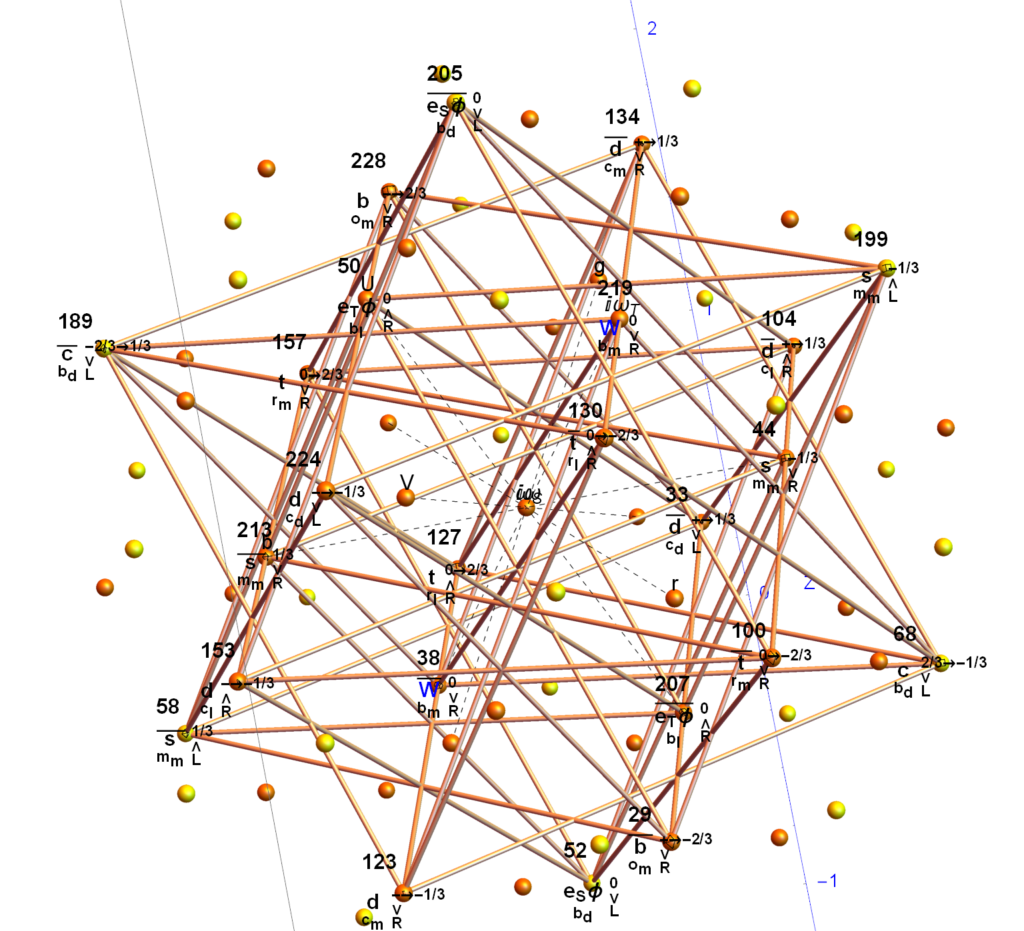The yellow spheres are those of the outer hull of singular 30 vertices of an icosidodecahedron. The orange spheres are pairs of icosahedron or dodecahedron vertices. The edges with numbered vertices form one of five 24-cells in the 120 vertex H4Φ scaled 600-cell within E8. Specifically, it is the 3rd rotation (of 4 π/5 rotations) within the 96 vertices of the snub-24-cell. You can see that 6 of the yellow vertices connect to the 24-cell. Rotating that 24-cell four times in 3-space by π/5 gives the connections to the rest of the vertices in H4Φ and completes the 30 vertex icosidodecahedron . The same is true for the 120 vertices of H4 using the corresponding 24-cell in H4.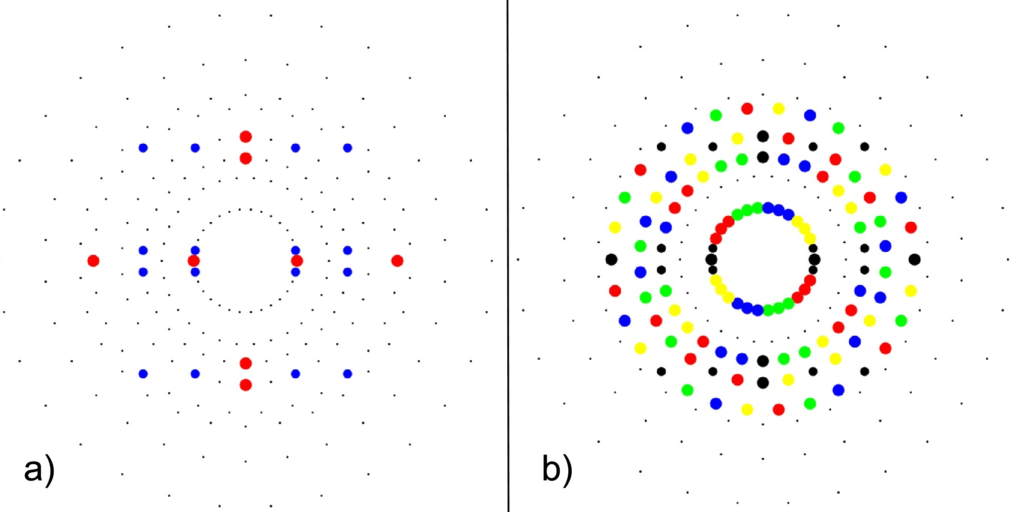a) 24-cell highlighting the 16-cell (red on-axis vertices) and 8-cell (blue off-axis vertices)b) Snub 24-cell highlighting four π/5 rotations of the 24-cell (black) in red, green, blue, yellow.

The following paper hulls-24cells-combined.pdf (13MB) & interactive Mathematica Notebook hulls-24cells-combined.nb (34MB) contains a comprehensive set of images that show the contents of the 7 concentric hulls of Platonic solid related shapes as well as their integration with one of the rotations of 24-cells in H4 and H4Φ in E8 (the same one used in the image above).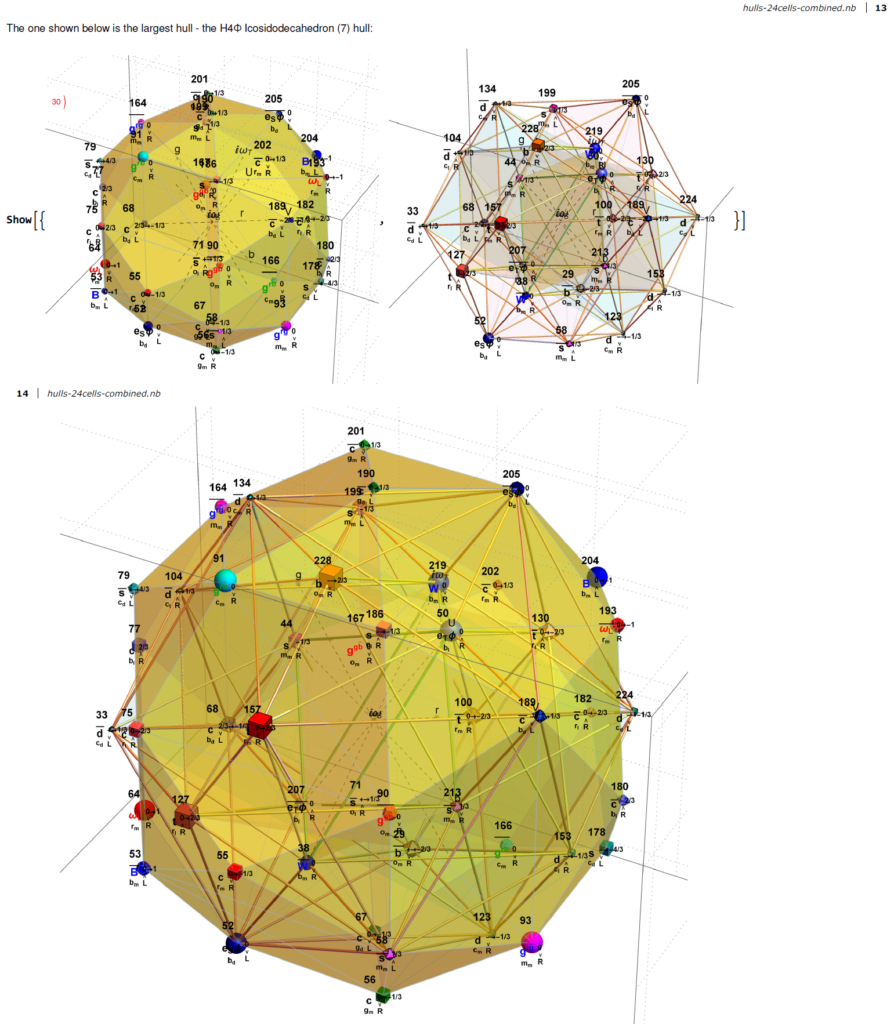Snapshot from the paper showing the 3rd rotation of the snub 24-cell and the icosidodecahedron of H4Φ

In the E8 Petrie projection, every hull (each with 2 or 4 overlapping vertices) pair into left/right patterns. See the set of four icosahedrons that occupy the 3rd hull in both 2D and 3D (the yellow edge sets belongs to the H4Φ and the blue sets belong to H4) :

The pair of dodecahedrons that occupy the 5th hull in both 2D and 3D (the yellow edge set belongs to the H4Φ and the blue belong to H4) :

Below is an animation that cycles through the sequence of 2D Petrie Projections of the pairs of hulls. One cycle shows each frame individually and the other builds the E8 Petrie from the previous 2D hull.

Below are images of the left (H4) and right (H4Φ) 2D Petrie projections of the hulls (which are defined by the Norm’s of 3D projected vertices using the E8->H4 folding matrix rows 2-4 as basis vectors). The two 30 vertex 4 & 7 Icosidodecahedron hulls and four 0th hull (points) are omitted leaving the gaps in the diagram. When these gap vertices are combined with the 48 3rd hull Icosahedrons (above), they make up the 112 integer D8 group assigned to the Bosons (48) and 2nd generation Fermions (64) in the physics model described below.

The combined set of hulls projected using the rows of the folding matrix as basis vectors is shown below from previous work:

This paper hull-list-3b.pdf (4MB) & interactive Mathematica Notebook hull-list-3b.nb (20MB) contains the visualizations and detail E8 vertices associated with each Platonic solid related concentric hulls. This paper 24cell-list-3b.pdf (6MB) & interactive Mathematica Notebook 24cell-list-3b.nb (20MB) contains the visualizations and detail for the five 24-cells in each H4 & H4Φ. Both of these papers also have detail information about its assigned physics particle based on a modified A.G. Lisi model (shown below). This paper describes these particle assignment symmetries in more detail.

# Latest Paper – Unimodular rotation of E8 to H4 600-cells

## Please see my latest paper that describes some advances in understanding the E8 to H4 rotation matrix

https://theoryofeverything.org/TOE/JGM/Unimodular-Rotation-of-E8-to-H4.pdf

Abstract: We introduce a unimodular Determinant=1 8×8 rotation matrix to produce four 4 dimensional copies of H4 600-cells from the 240 vertices of the Split Real Even E8 Lie group. Unimodularity in the rotation matrix provides for the preservation of the 8 dimensional volume after rotation, which is useful in the application of the matrix in various fields, from theoretical particle physics to 3D visualization algorithm optimization.

# Stereoscopic 3D Interactive Solar system simulation (shoemaker-Levy-9)

## It requires local installation of the free Mathematica CDF plugin.

This now includes the 1998 OR2 “Planet Killer” that will pass very near Earth in April 2020 (when selecting the Asteroid vs. Comet).

Now with improved UI, better planet scaling, and Anaglyph viz.

# Mathematica Analysis of Cohl Furey’s octonion and Clifford group theoretic ℂ⊗O assignments to standard model particles

I’ve read some recent papers by Cohl Furey and was intrigued by the potential relationship between the octonion and Clifford group theoretic assignments to standard model particles. Since I have developed an extensive Mathematica notebook to perform symbolic analysis using these structures (derived primarily for E8 Lie group work), I decided to follow her suggestion… “The reader is encouraged to check that C⊗O forms the 64-complex-dimensional Clifford algebra Cl(6), generated by the set {i e1,…i e6} acting on f”.

So to that end, I created the this .pdf with some preliminary results of that analysis. I found a few minor issues, but so far the model seems to symbolically compute consistently. Very cool!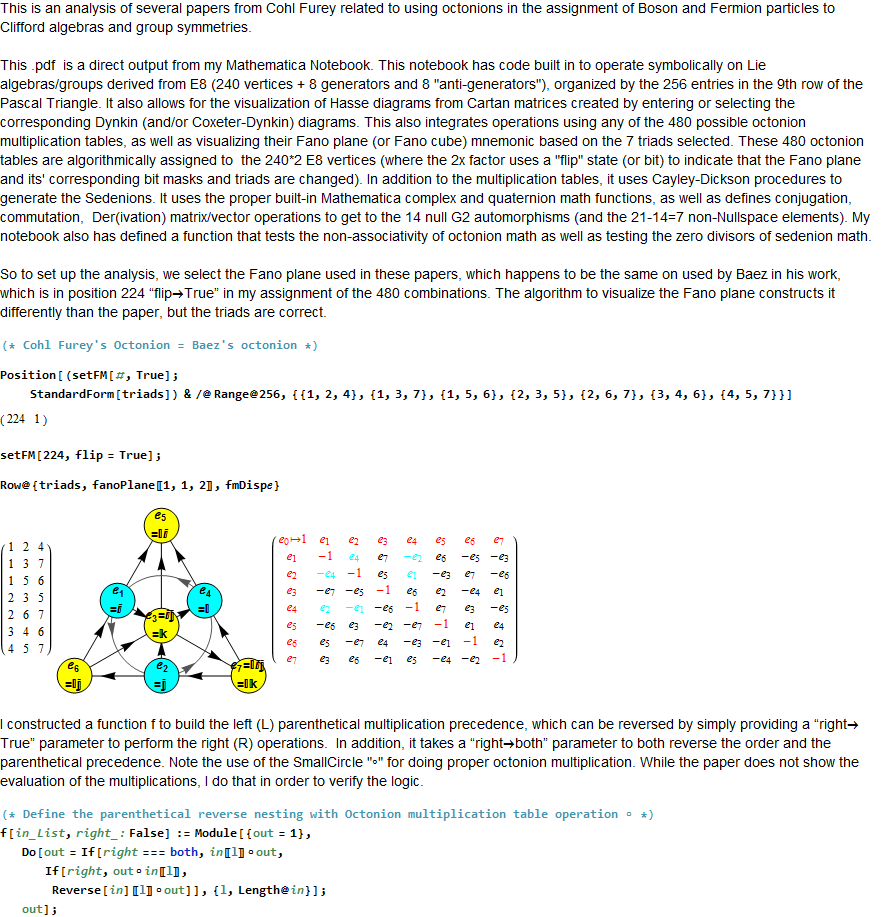# Latest paper: Even FibBinary Numbers and the Golden Ratio

Please see my latest paper here.

It adds information related to my previous blog post here.

Abstract: Previously, a determination of the relationship between the Natural numbers (N) and the n’th odd fibbinary number has been made using a relationship with the Golden ratio phi=(Sqrt+1}/2 and tau=1/phi. Specifically, if the n’th odd fibbinary equates to the j’th N, then j=Floor[n phi^2 – 1]. This note documents the completion of the relationship for the even fibbinary numbers, such that if the n’th even fibbinary equates to the j’th N, then j=Floor[n phi]-1, starting at j=0 for n=1. Alternatively, starting at j=2 for n=1, then j=Floor[n phi + tau].

Title:Even FibBinary Numbers and the Golden Ratio
Authors: J Gregory Moxness
Comments: Dec 31 2018, 5 pages, 4 Figures.
Subjects: Combinatorics

Below is a listing of the N integers represented by their 38 odd FibBinaries, along with the binary and Gray code Compositions.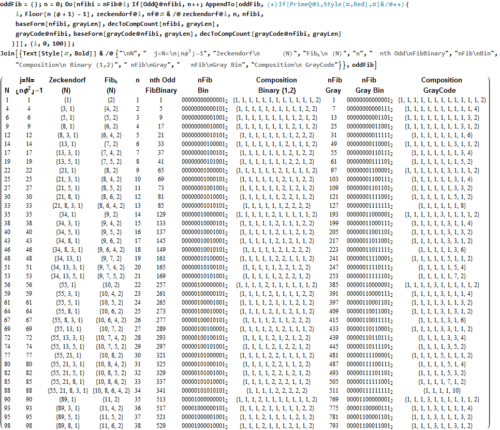Below is a listing of the first 63 N integers represented by their even FibBinaries, along with the binary and Gray code Compositions.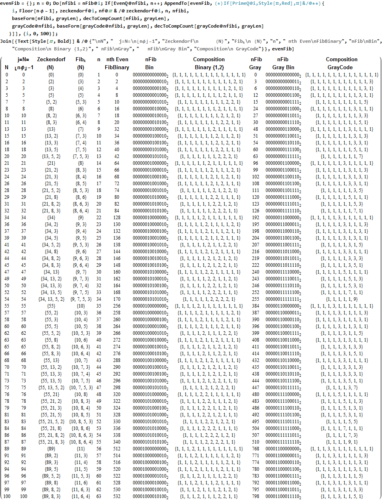Code output examples:Mathematica Code Snippets: